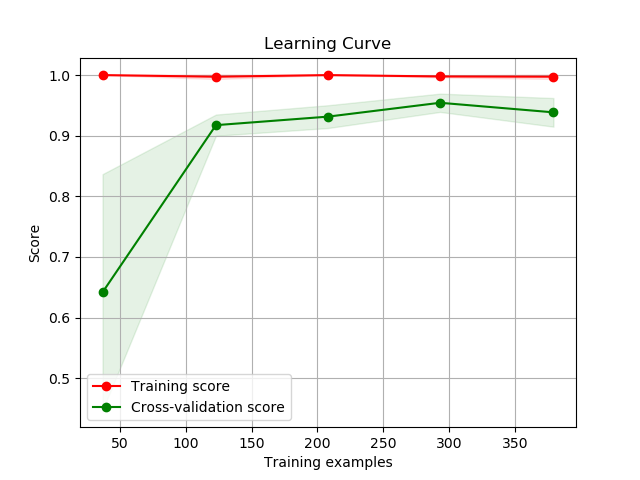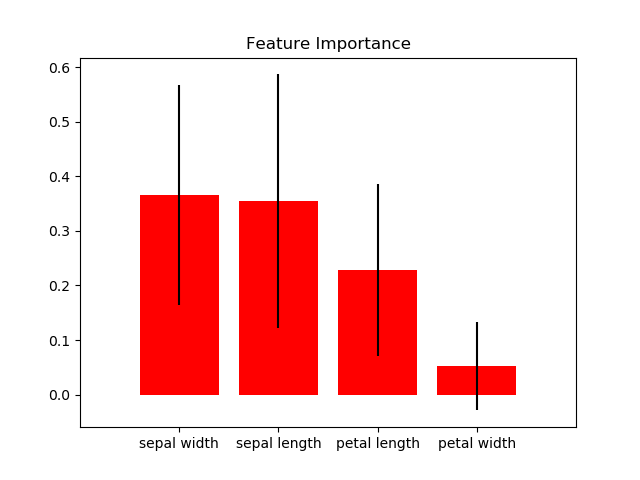# Estimators Module (API Reference)¶

The `scikitplot.estimators` module includes plots built specifically for scikit-learn estimator (classifier/regressor) instances e.g. Random Forest. You can use your own estimators, but these plots assume specific properties shared by scikit-learn estimators. The specific requirements are documented per function.

`scikitplot.estimators.``plot_learning_curve`(clf, X, y, title='Learning Curve', cv=None, shuffle=False, random_state=None, train_sizes=None, n_jobs=1, scoring=None, ax=None, figsize=None, title_fontsize='large', text_fontsize='medium')

Generates a plot of the train and test learning curves for a classifier.

Parameters: clf – Classifier instance that implements `fit` and `predict` methods. X (array-like, shape (n_samples, n_features)) – Training vector, where n_samples is the number of samples and n_features is the number of features. y (array-like, shape (n_samples) or (n_samples, n_features)) – Target relative to X for classification or regression; None for unsupervised learning. title (string, optional) – Title of the generated plot. Defaults to “Learning Curve” cv (int, cross-validation generator, iterable, optional) – Determines the cross-validation strategy to be used for splitting. Possible inputs for cv are: None, to use the default 3-fold cross-validation, integer, to specify the number of folds. An object to be used as a cross-validation generator. An iterable yielding train/test splits. For integer/None inputs, if `y` is binary or multiclass, `StratifiedKFold` used. If the estimator is not a classifier or if `y` is neither binary nor multiclass, `KFold` is used. shuffle (bool, optional) – Used when do_cv is set to True. Determines whether to shuffle the training data before splitting using cross-validation. Default set to True. random_state (int `RandomState`) – Pseudo-random number generator state used for random sampling. train_sizes (iterable, optional) – Determines the training sizes used to plot the learning curve. If None, `np.linspace(.1, 1.0, 5)` is used. n_jobs (int, optional) – Number of jobs to run in parallel. Defaults to 1. scoring (string, callable or None, optional) – default: None A string (see scikit-learn model evaluation documentation) or a scorerbcallable object / function with signature scorer(estimator, X, y). ax (`matplotlib.axes.Axes`, optional) – The axes upon which to plot the curve. If None, the plot is drawn on a new set of axes. figsize (2-tuple, optional) – Tuple denoting figure size of the plot e.g. (6, 6). Defaults to `None`. title_fontsize (string or int, optional) – Matplotlib-style fontsizes. Use e.g. “small”, “medium”, “large” or integer-values. Defaults to “large”. text_fontsize (string or int, optional) – Matplotlib-style fontsizes. Use e.g. “small”, “medium”, “large” or integer-values. Defaults to “medium”. The axes on which the plot was drawn. ax (`matplotlib.axes.Axes`)

Example

```>>> import scikitplot as skplt
>>> rf = RandomForestClassifier()
>>> skplt.estimators.plot_learning_curve(rf, X, y)
<matplotlib.axes._subplots.AxesSubplot object at 0x7fe967d64490>
>>> plt.show()
````scikitplot.estimators.``plot_feature_importances`(clf, title='Feature Importance', feature_names=None, max_num_features=20, order='descending', x_tick_rotation=0, ax=None, figsize=None, title_fontsize='large', text_fontsize='medium')

Generates a plot of a classifier’s feature importances.

Parameters: clf – Classifier instance that has a `feature_importances_` attribute, e.g. `sklearn.ensemble.RandomForestClassifier` or `xgboost.XGBClassifier`. title (string, optional) – Title of the generated plot. Defaults to “Feature importances”. feature_names (None, `list` of string, optional) – Determines the feature names used to plot the feature importances. If None, feature names will be numbered. max_num_features (int) – Determines the maximum number of features to plot. Defaults to 20. order ('ascending', 'descending', or None, optional) – Determines the order in which the feature importances are plotted. Defaults to ‘descending’. x_tick_rotation (int, optional) – Rotates x-axis tick labels by the specified angle. This is useful in cases where there are numerous categories and the labels overlap each other. ax (`matplotlib.axes.Axes`, optional) – The axes upon which to plot the curve. If None, the plot is drawn on a new set of axes. figsize (2-tuple, optional) – Tuple denoting figure size of the plot e.g. (6, 6). Defaults to `None`. title_fontsize (string or int, optional) – Matplotlib-style fontsizes. Use e.g. “small”, “medium”, “large” or integer-values. Defaults to “large”. text_fontsize (string or int, optional) – Matplotlib-style fontsizes. Use e.g. “small”, “medium”, “large” or integer-values. Defaults to “medium”. The axes on which the plot was drawn. ax (`matplotlib.axes.Axes`)

Example

```>>> import scikitplot as skplt
>>> rf = RandomForestClassifier()
>>> rf.fit(X, y)
>>> skplt.estimators.plot_feature_importances(
...     rf, feature_names=['petal length', 'petal width',
...                        'sepal length', 'sepal width'])
<matplotlib.axes._subplots.AxesSubplot object at 0x7fe967d64490>
>>> plt.show()
```